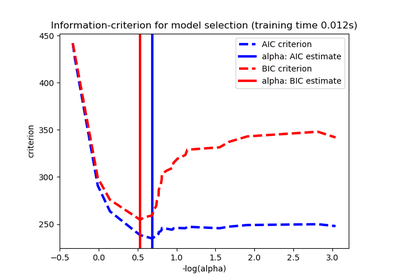# 3.2.4.2.1. `sklearn.linear_model`.LassoLarsIC¶

class `sklearn.linear_model.``LassoLarsIC`(criterion=’aic’, fit_intercept=True, verbose=False, normalize=True, precompute=’auto’, max_iter=500, eps=2.220446049250313e-16, copy_X=True, positive=False)[source]

Lasso model fit with Lars using BIC or AIC for model selection

The optimization objective for Lasso is:

```(1 / (2 * n_samples)) * ||y - Xw||^2_2 + alpha * ||w||_1
```

AIC is the Akaike information criterion and BIC is the Bayes Information criterion. Such criteria are useful to select the value of the regularization parameter by making a trade-off between the goodness of fit and the complexity of the model. A good model should explain well the data while being simple.

Read more in the User Guide.

Parameters: criterion : ‘bic’ | ‘aic’ The type of criterion to use. fit_intercept : boolean whether to calculate the intercept for this model. If set to false, no intercept will be used in calculations (e.g. data is expected to be already centered). verbose : boolean or integer, optional Sets the verbosity amount normalize : boolean, optional, default True This parameter is ignored when `fit_intercept` is set to False. If True, the regressors X will be normalized before regression by subtracting the mean and dividing by the l2-norm. If you wish to standardize, please use `sklearn.preprocessing.StandardScaler` before calling `fit` on an estimator with `normalize=False`. precompute : True | False | ‘auto’ | array-like Whether to use a precomputed Gram matrix to speed up calculations. If set to `'auto'` let us decide. The Gram matrix can also be passed as argument. max_iter : integer, optional Maximum number of iterations to perform. Can be used for early stopping. eps : float, optional The machine-precision regularization in the computation of the Cholesky diagonal factors. Increase this for very ill-conditioned systems. Unlike the `tol` parameter in some iterative optimization-based algorithms, this parameter does not control the tolerance of the optimization. copy_X : boolean, optional, default True If True, X will be copied; else, it may be overwritten. positive : boolean (default=False) Restrict coefficients to be >= 0. Be aware that you might want to remove fit_intercept which is set True by default. Under the positive restriction the model coefficients do not converge to the ordinary-least-squares solution for small values of alpha. Only coefficients up to the smallest alpha value (```alphas_[alphas_ > 0.].min()``` when fit_path=True) reached by the stepwise Lars-Lasso algorithm are typically in congruence with the solution of the coordinate descent Lasso estimator. As a consequence using LassoLarsIC only makes sense for problems where a sparse solution is expected and/or reached. coef_ : array, shape (n_features,) parameter vector (w in the formulation formula) intercept_ : float independent term in decision function. alpha_ : float the alpha parameter chosen by the information criterion n_iter_ : int number of iterations run by lars_path to find the grid of alphas. criterion_ : array, shape (n_alphas,) The value of the information criteria (‘aic’, ‘bic’) across all alphas. The alpha which has the smallest information criterion is chosen. This value is larger by a factor of `n_samples` compared to Eqns. 2.15 and 2.16 in (Zou et al, 2007).

Notes

The estimation of the number of degrees of freedom is given by:

“On the degrees of freedom of the lasso” Hui Zou, Trevor Hastie, and Robert Tibshirani Ann. Statist. Volume 35, Number 5 (2007), 2173-2192.

Examples

```>>> from sklearn import linear_model
>>> reg = linear_model.LassoLarsIC(criterion='bic')
>>> reg.fit([[-1, 1], [0, 0], [1, 1]], [-1.1111, 0, -1.1111])
...
LassoLarsIC(copy_X=True, criterion='bic', eps=..., fit_intercept=True,
max_iter=500, normalize=True, positive=False, precompute='auto',
verbose=False)
>>> print(reg.coef_)
[ 0.  -1.11...]
```

Methods

 `fit`(X, y[, copy_X]) Fit the model using X, y as training data. `get_params`([deep]) Get parameters for this estimator. `predict`(X) Predict using the linear model `score`(X, y[, sample_weight]) Returns the coefficient of determination R^2 of the prediction. `set_params`(**params) Set the parameters of this estimator.
`__init__`(criterion=’aic’, fit_intercept=True, verbose=False, normalize=True, precompute=’auto’, max_iter=500, eps=2.220446049250313e-16, copy_X=True, positive=False)[source]
`fit`(X, y, copy_X=True)[source]

Fit the model using X, y as training data.

Parameters: X : array-like, shape (n_samples, n_features) training data. y : array-like, shape (n_samples,) target values. Will be cast to X’s dtype if necessary copy_X : boolean, optional, default True If `True`, X will be copied; else, it may be overwritten. self : object returns an instance of self.
`get_params`(deep=True)[source]

Get parameters for this estimator.

Parameters: deep : boolean, optional If True, will return the parameters for this estimator and contained subobjects that are estimators. params : mapping of string to any Parameter names mapped to their values.
`predict`(X)[source]

Predict using the linear model

Parameters: X : {array-like, sparse matrix}, shape = (n_samples, n_features) Samples. C : array, shape = (n_samples,) Returns predicted values.
`score`(X, y, sample_weight=None)[source]

Returns the coefficient of determination R^2 of the prediction.

The coefficient R^2 is defined as (1 - u/v), where u is the residual sum of squares ((y_true - y_pred) ** 2).sum() and v is the total sum of squares ((y_true - y_true.mean()) ** 2).sum(). The best possible score is 1.0 and it can be negative (because the model can be arbitrarily worse). A constant model that always predicts the expected value of y, disregarding the input features, would get a R^2 score of 0.0.

Parameters: X : array-like, shape = (n_samples, n_features) Test samples. y : array-like, shape = (n_samples) or (n_samples, n_outputs) True values for X. sample_weight : array-like, shape = [n_samples], optional Sample weights. score : float R^2 of self.predict(X) wrt. y.
`set_params`(**params)[source]

Set the parameters of this estimator.

The method works on simple estimators as well as on nested objects (such as pipelines). The latter have parameters of the form `<component>__<parameter>` so that it’s possible to update each component of a nested object.

Returns: self :

## 3.2.4.2.1.1. Examples using `sklearn.linear_model.LassoLarsIC`¶Lasso model selection: Cross-Validation / AIC / BIC